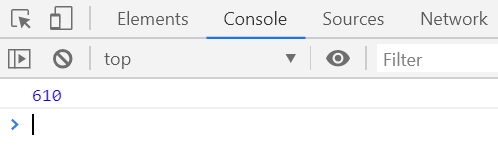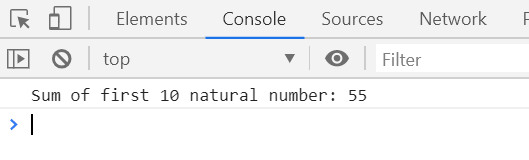Open in App
Not now

# Underscore.js _.memoize() Function

• Last Updated : 24 Nov, 2021

The _.memoize() function is used to memorize a given function by caching the result computed by the function. It is used to speed up for the slow running process.

Syntax:

`_.memoize(function, [hashFunction])`

Parameters: This function accepts two parameters as mentioned above and described below:

• function: The function that need to be executed.
• hashFunction: It is an optional parameter. The hashFunction is used to compute the hash key for storing the result.

Return Value: It returns the result of the called function.

Below examples illustrate the _.memoize function in Underscore.js:
Example 1:

 ` ` `<``html``> ` ` `  `<``head``> ` `    ``<``script` `type``=``"text/javascript"` `src``= ` `"https://cdnjs.cloudflare.com/ajax/libs/underscore.js/1.9.1/underscore-min.js"``> ` `    `` ` ` ` ` `  `<``body``> ` `    ``<``script` `type``=``"text/javascript"``> ` ` `  `        ``var fib = _.memoize(function (n) { ` `            ``return n < ``2` `? n : fib(n - 1) + fib(n - 2); ` `        ``}); ` ` `  `        ``console.log(fib(15)); ` `    `` ` ` ` ` `  ` `

Output:Example 2:

 ` ` `<``html``> ` ` `  `<``head``> ` `    ``<``script` `type``=``"text/javascript"` `src``= ` `"https://cdnjs.cloudflare.com/ajax/libs/underscore.js/1.9.1/underscore-min.js"``> ` `    `` ` ` ` ` `  `<``body``> ` `    ``<``script` `type``=``"text/javascript"``> ` ` `  `        ``var sum = _.memoize(function (n) { ` `            ``return n < ``1` `? n : n + sum(n - 1); ` `        ``}); ` ` `  `        ``console.log('Sum of first 10 natural number: ' + sum(10)); ` `    `` ` ` ` ` `  ` `

Output:My Personal Notes arrow_drop_up
Related Articles